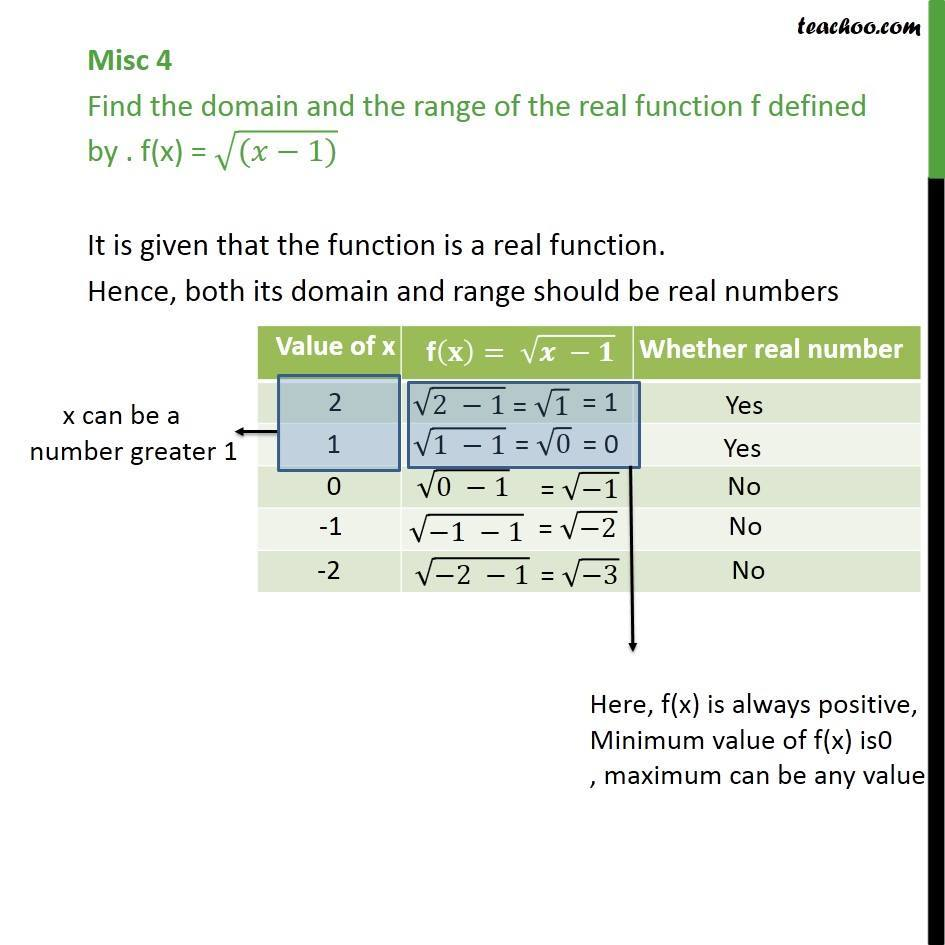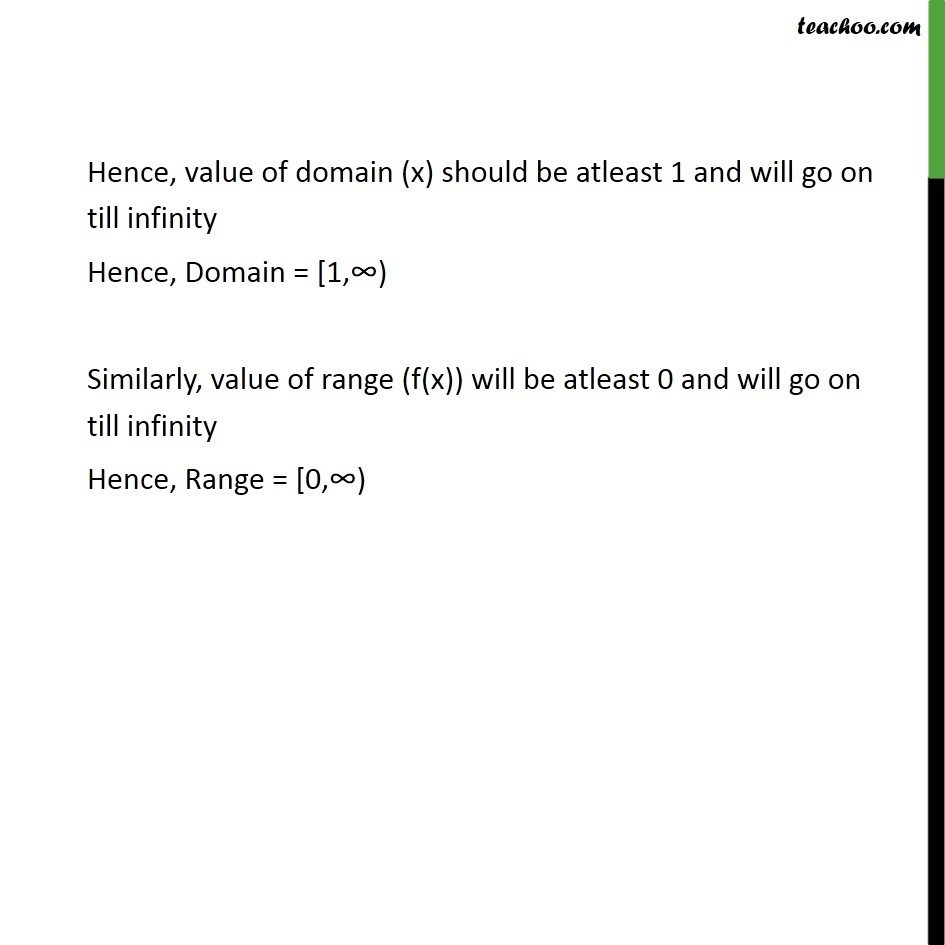1. Chapter 2 Class 11 Relations and Functions
2. Serial order wise
3. Miscellaneous

Transcript

Misc 4 Find the domain and the range of the real function f defined by . f(x) = (( 1)) It is given that the function is a real function. Hence, both its domain and range should be real numbers Hence, value of domain (x) should be atleast 1 and will go on till infinity Hence, Domain = [1, ) Similarly, value of range (f(x)) will be atleast 0 and will go on till infinity Hence, Range = [0, )

Miscellaneous